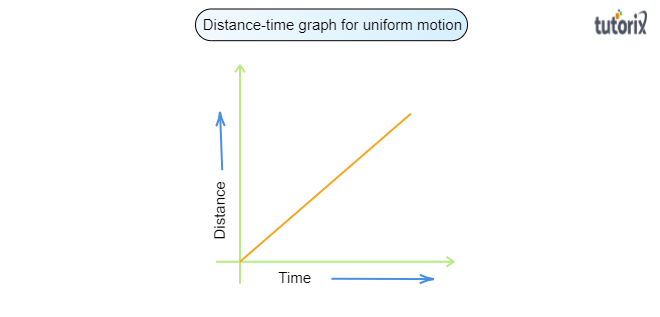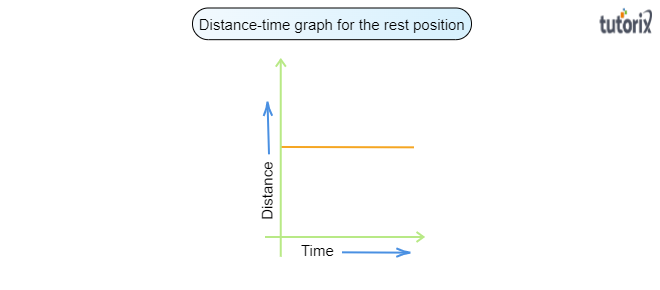# Show the shape of the distance-time graph for the motion in the following cases:$(i)$. A car moving with a constant speed.$(ii)$. A car parked on a side road.

To do:

To show the shape of the distance-time graph for the motion in the following cases:

$(i)$. A car moving with a constant speed.

$(ii)$. A car parked on a side road.

Solution:

Before drawing and showing the shape of the graph of a moving car with a constant speed and a car parked on a side road, we will know the motion of the both the cars. Let us discuss the motion of a moving car with a constant speed and a car parked on a side road:

Motion of a car moving with a constant speed:

When a car is moving with a constant speed, its motion is called uniform motion and it will cover a equal distances in equal interval of time.

Motion of a car parked on a side road:

When a car is parked on a side road, there is no motion. The car parked in a side road is in the rest position.

$(i)$. Distance-time graph for a car moving with a constant speed:

As we have discussed above that a car moving with a constant speed shows exhibits a uniform motion and covers equal distances in equal time intervals. On plotting the covered equal distances and the corresponding equal time intervals on a graph we obtain a straight line as the distance-time graph shown below:$(ii)$. Distance-time graph for a car parked on a side road:

The car parked in a side road is in the rest position. There is no distance covered by parked car with respect to the time. On plotting a the distance-time graph for the motion of a car parked on a side road is as below: